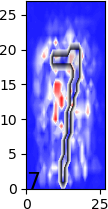## Thursday, November 29, 2018

### Explainable AI (XAI): Sensitivity Analysis

Imagine you have a neural network that can classify images well. At test time, would it be enough to just know what the class of the image is? Or would you also want to know why the image was classified the way it was? This is one of the goals of explainable AI and in this post we'll see what sensitivity analysis is.

Sensitivity analysis is a way to measure the importance of different parts of an input to one part of an output. In other words, you want to know which pixels were most important to give a high probability for a particular class. The way sensitivity analysis measures this is by measuring how sensitive the output is to each pixel, that is, which pixels, when changed, will change the output the most. Most pixels should leave the output unchanged when they themselves are changed, but some pixels would be critical to the particular output that the neural network has given. To measure this, we simply find the following:

$\left|\frac{df(x)_i}{dx_j}\right|$

that is, the sensitivity of output $i$ to input $j$ is the absolute value of the gradient of the output with respect to the input. In Tensorflow we can compute this as follows:

sensitivity = tf.abs(tf.gradients([outputs[0, output_index]], [images]))

where 'output_index' is a placeholder that is a scalar of type tf.int64, 'outputs' is a softmax with probabilities for each class and where the first index is the batch index and the second index is the class probability, and 'images' is the pixels of images where the first index is batch index and the second index is the image in vector form. This code also assumes that only the first image in the batch is to be analysed. This is because Tensorflow can only find the gradient of a single scalar so we can only find the sensitivity of a single output of a single image.

Here are some examples I got when I tried it on a simple fully connected two layer neural network trained to classify MNIST handwritten digits.These heat maps show which pixels have the highest sensitivity score for the digit they were classified as. We can see how the empty space in the middle is important for classifying the zero. The four and the nine can be easy confused for each other were it not for the little bit in the top left corner. The seven can be confused for a nine if we complete the top left loop and the five can be confused with a six or eight if we draw a little diagonal line.